# Quiz 7: Production Economics

The production function is given as: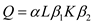We need to calculate the parameters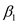and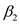. We will use the regression analysis.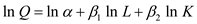We denote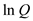by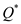, ln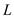by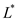and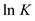by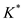. Therefore,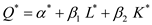In the table below, we will calculate the values of,,,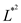,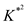,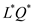,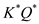and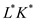.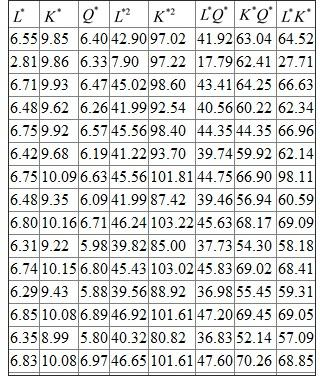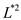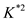The Cobb-Douglas production function can be written as:a) In this table, we will calculate the marginal product and average product. The total product is given.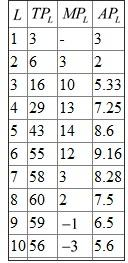The capital is fixed at 500-bhp rating. The total product of laboris output. The marginal product of labor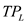is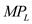. The average product of labor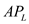is total product divided by labor. In this question, we were given the total product. We were to calculate the average product and the marginal product. For instance, the total product at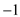is 16. Hence the average product at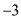is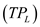. In a similar way, we can calculate the average product for all labor inputs. The total product at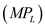is 6 and at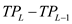is 16. The marginal product at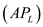is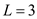. Similarly, we can calculate the marginal product for other labor inputs. b) We will draw the total product, average product and marginal product curve here.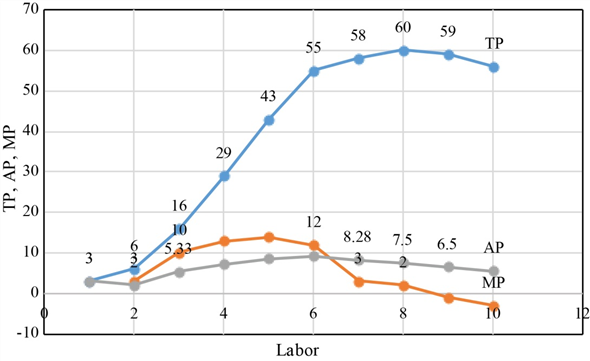The TP, MP and AP are shown in the diagram above. The point to note is that when average product is at its maximum point, it is equal to the marginal product curve. c) In stage 1, both AP and MP are rising. MP reaches its maximum point and starts falling. The point to note is that MP is always greater than AP in this stage. In stage 2, AP reaches its maximum point and starts falling. MP also falls and becomes zero. Here MP is always less than AP. In stage 3, MP becomes negative and AP decreases but remains positive.
The cobb-Douglas production function takes the form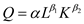Where L is the labor input and K is the capital input used in producing Q units of output, and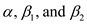are the constants. Take log on both sides of the equation to derive following.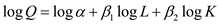The table below shows the output levels with varying units of labor and capital and their logs. • Calculate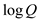by entering the formula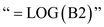in cell E2 and stretching it through cell E16. • Calculate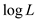by entering the formula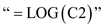in cell F2 and stretching it through cell F16. • Calculate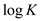by entering the formula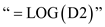in cell G2 and stretching it through cell G16.To run regression, • Select the "Data" tab and click "Data Analysis" in the "Analysis" grouping. • Input the data of dependent variable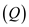in the "Input Y-Range" field, and then enter data of independent variables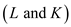in the "Input X-Range" field for multiple columns. • Click "OK" to create the analysis as below.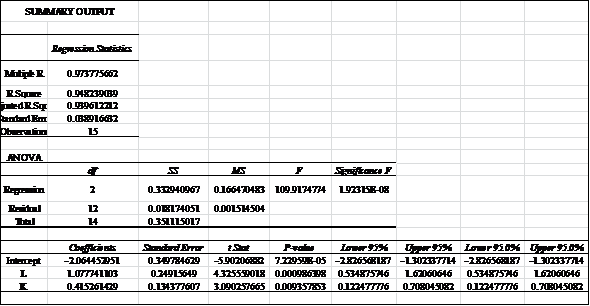The intercept is calculated as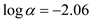. The coefficients of labor and capital are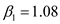and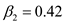, respectively. Thus, the regression equation estimated using OLS is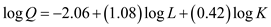. Since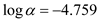, thus,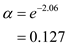Thus, the estimated equation in its multiplicative form is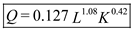. We want to test the hypothesis that whether units of labor and capital are useful in predicting the output at 0.05 level of significance. That is, we would perform a statistical test to determine whether the sample valuesandare significantly different from zero. For Labor: The null hypothesis is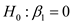, no relationship between output and labor. And, the alternative hypothesis is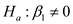, no relationship between output and labor. With 15 observations, a t -distribution for the sample statisticwill have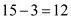degrees of freedom. From Table 2 of Appendix B, the t-value is obtained as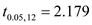The calculated value of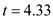is greater than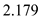, we reject null hypothesis. Thus, we conclude relationship exists between output and labor at the 5 percent level of significance. For Capital: The null hypothesis is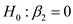, no relationship between output and capital. And, the alternative hypothesis is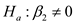, no relationship between output and capital. With 15 observations, a t -distribution for the sample statisticwill havedegrees of freedom. From Table 2 of Appendix B, the t-value is obtained asThe calculated value of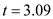is greater than, we reject null hypothesis. Thus, we conclude relationship exists between output and capital at the 5 percent level of significance.